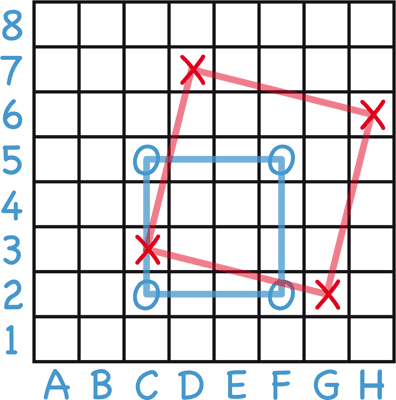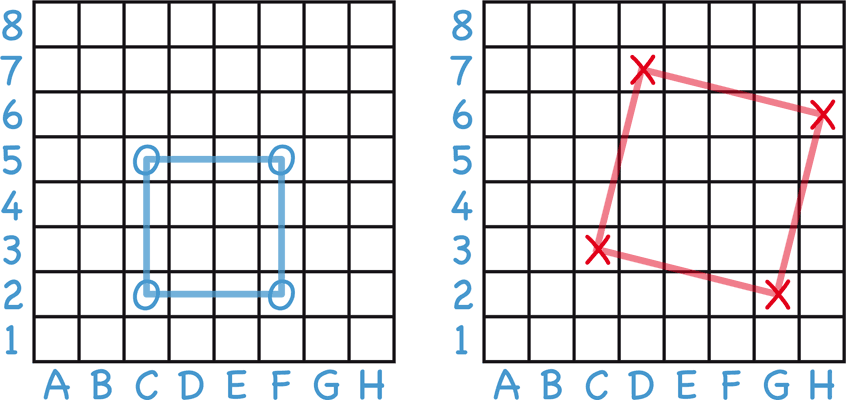## MetaSquares

Players: Two

Each player scores points for making a square with four of their pieces.

### Description

One player is 'O' and the other is 'X'. The players take turns in drawing their symbol in one of the cells on a 8 x 8 grid. When a player gets four pieces at the corners of a square, the square is drawn in and the player scores points for the square:Squares may be any size or slanted at any angle, but must be a perfect square.

The winner is the first player to reach a target number of points.

### Simple game

The simple game avoids the complexity of the scoring system, and players score one point for each square they draw, whatever its area.

On an 8 x 8 board the winner is the first player to complete 8 squares.

In the advanced game each player scores according to the area of each square they create.

On an 8 x 8 board the winner is the first player to reach 150 points and be 15 or more points ahead of their opponent.

The score for completing a square is calculated by multiplying the number of cells along each side by itself.

For example, the blue square below has 4 cells along each edge, so this scores 4 x 4 or 16 points.

For slanted squares the length of each side is taken as the number of cells along the 'L' between two adjacent corners. For the red square below this is six cells, giving a score of 6 x 6 or 36:Example

History